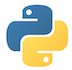Related Tags

python
cheat sheet
syntax
beginner

# Python 3 cheat sheetEducative Answers Team

The purpose of this Python 3 cheat sheet is to help beginners remember Python syntax.## Primitive data types and variable assignment

# The primitive data types are integer, float, boolean and string

# assigning values to primitives
int_var = 2
print("integer:", int_var)
float_var = 1.9
print("float:", float_var)
bool_var = True
print("boolean:", bool_var)
string_var = 'Hello world'
print("string:", string_var)

# icrementing a variable's value
int_var += 1
print("integer:", int_var)

# x, y and z all get the value 0.1
x = y = z = 0.1
print("x:", x, "y:", y, "z:", z)

# u gets the value 100 and v gets the value 12
u, v = 100, 12
print("u:", v, "v:", v)

## Conditional statements

country = 'South Korea'

if country == 'South Korea':
print('Anneyong')
elif country == 'France':
print('Bonjour')
else:
print('Hello')

## Loops

# printing the 0, 1
print("Example 1:")
for i in range(2):
print(i)

# printing numbers from 0 to 2 in the reverse order
print("Example 2:")
i = 2
while i >= 0:
print(i)
i -= 1

# printing all the characters in a string until a
# space is encountered is encountered
print("Example 3:")
s = 'Hey World'
for character in s:
if character == ' ':
# The break keyword is used to exit the loop.
break
else:
print(character)

# printing all the characters in a string except for any space.
print("Example 4:")
s = 'Tic Tac'
for character in s:
if character == ' ':
# The continue keyword is used to ignore the subsequent
# lines of code in the loop for this iteration and start the
# next iteration.
continue
print(character)

## Functions

# a function may or may not return anything
def multiply(num1, num2):
return num1 * num2
print(multiply(3, 4))

# a function can accept variable number of arguments as well
def multipleargs(*args):
print(args)
multipleargs('a', 'b', 'c', 'd')

## Classes and objects

class Fruit:
#__init__() is always executed when the class is being initiated
def __init__(self, name, weight):
self.name = name
self.weight = weight
# a method of the class
def print_fruit(self):
print("Name:", self.name, "Weight(g):", self.weight)

# Creating an object
fruit1 = Fruit("Kiwi", 60)

# calling the print_fruit() method
fruit1.print_fruit()

## Lists

l = [1, 2 ,3]

# adding an element to the end of the list
l.append(4)
print(l)

# adding element -1 at index 3
l.insert(3,-1)
print(l)

# adding element -1 at index 1
l.insert(1,-1)
print(l)

# removing first item with value -1
l.remove(-1)
print(l)

# remove and return the element at index 2
elem = l.pop(2)
print("element popped:", elem)
print(l)

# sorting a list
l.sort()
print(l)

# printing the list starting from index 0 and ending at index 2
print(l[0:3])

# printing the second last element of the list
print(l[-2])

# List comprehension.
# converting all the elements of the list to uppercase
print([x.upper() for x in ["x","y","z"]])

# List of lists
m = [['kiwi','watermelon'],['20','30','40']]
print(m)

## Dictionaries

fruit =	{
"name": "Mango",
"weight": 60,
"origin": "Pakistan"
}

# Printing the dictionary
print(fruit)

# Using a key to look up value
print(fruit["name"])

# Changing the value of an item
fruit["name"] = "Banana"
print(fruit["name"])

fruit["color"] = "Yellow"
print(fruit)

RELATED TAGS

python
cheat sheet
syntax
beginner